### Eight-node axisymmetric element (CAX8 and CAX8R)

This is a general purpose quadratic axisymmetric element. Just as the shell, plane stress and plane strain element it is internally expanded into a C3D20 or C3D20R element according to Figure 69 and the node numbering of Figure 68 applies.

For axisymmetric elements the coordinates of the nodes correspond to the radial direction (first coordinate) and the axial direction (second or y-coordinate). The axisymmetric structure is expanded by rotation about the second coordinate axis, half clockwise and half counterclockwise. The radial direction corresponds to the x-axis in the 3D expansion, the axial direction with the y-axis. The x-y plane cuts the expanded structure in half. The z-axis is perpendicular to the x-y plane such that a right-hand-side axis system is obtained.

The same rules apply as for the plane strain elements, except that in-plane conditions in a plane strain construction now correspond to radial plane conditions in the axisymmetric structure. Expressed in another way, the z-direction in plane strain now corresponds to the circumferential direction in a cylindrical coordinate system with the y-axis as defining axis. Notice that nodes on the x-axis are not automatically fixed in radial direction. The user has to take care of this by using the *BOUNDARY card

Compared to plane strain elements, the following conditions apply:

• The expansion angle is fixed, its size is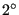. The value on the line beneath the *SOLID SECTION keyword, if any, has no effect.
• The displacements in cylindrical coordinates of all nodes not in the defining plane are identical to the displacements of the corresponding nodes in the defining plane. This is formulated using MPC's.
• Forces act in radial planes. They have to be defined for the complete circumference, i.e. if you apply a force in a node, you first have to sum all forces at that location along the circumference and then apply this sum to the node.
• Concentrated heat fluxes act in radial planes. They have to be defined for the complete circumference.
• Mass flow rates act in radial planes. They have to be defined for the complete circumference.
• For distributed loading Figure 71 applies.

A special application is the combination of axisymmetric elements with plane stress elements to model quasi-axisymmetric structures. Consider a circular disk with holes along the circumference, Figure 72. Assume that the holes take up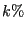of the circumferential width, i.e. if the center of the holes is located at a radius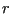, the holes occupy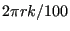. Then, the structure is reduced to a two-dimensional model by simulating the holes by plane stress elements with width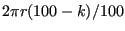and everything else by axisymmetric elements. More sophisticated models can be devised (e.g. taking the volume of the holes into account instead of the width at their center, or adjusting the material properties as well ). The point here is that due to the expansion into three-dimensional elements a couple of extra guidelines have to be followed: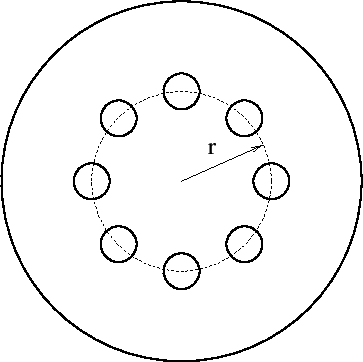• expanded plane stress and axisymmetric elements must have a small thickness to yield good results: in the case of plane stress elements this is because a large thickness does not agree with the plane stress assumption, in the case of axisymmetric elements because large angles yield bad results since the expansion creates only one layer of elements. CalculiX uses an expansion angle of, which amounts to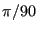radians. Consequently, only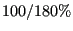of the disk is modeled and the thickness of the plane stress elements is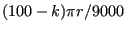. This is done automatically within CalculiX. On the *SOLID SECTION card the user must specify the thickness of the plane stress elements for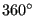, i.e..
• the point forces on the axisymmetric elements are to be given for the complete circumference, as usual for axisymmetric elements.
• the point forces on the plane stress elements act on the complete circumference.
• distributed loads are not affected, since they act on areas and/or volumes.

If an axisymmetric element is connected to a structure consisting of 3D elements the motion of this structure in the circumferential direction is not restricted by its connection to the 2D elements. The user has to take care that any rigid body motion of the structure involving the circumferential direction is taken care of, if appropriate. This particularly applies to any springs connected to axisymmetric elements.

Notice that structures containing axisymmetric elements should be defined in the global x-y plane, i.e. z=0 for all nodes.